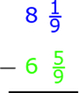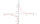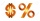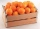# Evaluate mixed expressions

Which of the following is equal to 4 and 2 over 3 divided by 3 and 1 over 2?

A. 4 and 2 over 3 times 3 and 2 over 1
B. 14 over 3 times 2 over 7
C. 14 over 3 times 7 over 2
D. 42 over 3 times 2 over 31

Result

x = (Correct answer is: B)### Step-by-step explanation:Did you find an error or inaccuracy? Feel free to write us. Thank you!Tips to related online calculators
Need help to calculate sum, simplify or multiply fractions? Try our fraction calculator.

## Related math problems and questions:

• Fractions and mixed numerals(a) Convert the following mixed numbers to improper fractions. i. 3 5/8 ii. 7 7/6 (b) Convert the following improper fraction to mixed number. i. 13/4 ii. 78/5 (c) Simplify these fractions to their lowest terms. i. 36/42 ii. 27/45 2. evaluate following ex
• Simplify 7Simplify. 7-1/3÷ 3-2/3 of 2+ 4-1/2÷ 2-1/4+ 1/2 solution and by step by step
• Equivalent fractions 2Write the equivalent multiplication expression. 2 1/6÷3/4
• Evaluate valueEvaluate value of the following expressions: 1. The product of nine and a number 12, decreased by six 2. Eight decreased by three times a number 2. 3. The quotient of twice a number 49 and seven.
• ReciprocalsWhich among the given reciprocal is correct a. 3/15x1/3= 1 b. 3/20x20/3=1 c. 7/14x7/7=1 d. 34/3x34/34=1
• Divide 11Divide the product of 4 and 5/8 by 1 1/2. Write your answer as a mixed number.
• EvaluateThe division of numbers $a and$b increase by-product of the numbers $c and$d
• 76% is76% is between which of the following two numbers. 1) 1/5 & 1/4. 2) 1/3 & 1/2 3) 2/3 & 7/10 4) 3/4 & 5/6
• Numbers divisionWith what number should be divided mixed number 2 3/4 to get 11/12?
• Simplest form of a fractionWhich one of the following fraction after reducing in simplest form is not equal to 3/2? a) 15/20 b) 12/8 c) 27/18 d) 6/4
• Expressions with variableThis is algebra. Let n represent an unknown number and write the following expressions: 1. 4 times the sum of 7 and the number x 2. 4 times 7 plus the number x 3. 7 less than the product of 4 and the number x 4. 7 times the quantity 4 more than the number
• Evaluate 17Evaluate 2x+6y when x=- 4/5 and y=1/3. Write your answer as a fraction or mixed number in simplest form.
• Three digits number 2Find the number of all three-digit positive integers that can be put together from digits 1,2,3,4 and which are subject to the same time has the following conditions: on one positions is one of the numbers 1,3,4, on the place of hundreds 4 or 2.
• Difference mixed fractionsWhat is the difference between 4 2/3 and 3 1/6?
• Dividing walnuts into cratesThere are 8 and 2 over 3 pounds of walnuts in a container, which will be divided equally into containers that hold 1 and 1 over 5 pounds. This would fill n and 4 over 18 containers. What is n?
• Evaluate fractionsThe difference  of 5 1/2 and 2/3 is added to the product of 5/6 and 1/2Add two mixed fractions: 2 4/6 + 1 3/6